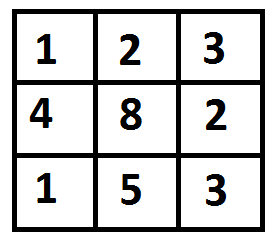C Program for Min Cost Path

• Difficulty Level : Medium
• Last Updated : 04 Dec, 2018

Given a cost matrix cost[][] and a position (m, n) in cost[][], write a function that returns cost of minimum cost path to reach (m, n) from (0, 0). Each cell of the matrix represents a cost to traverse through that cell. Total cost of a path to reach (m, n) is sum of all the costs on that path (including both source and destination). You can only traverse down, right and diagonally lower cells from a given cell, i.e., from a given cell (i, j), cells (i+1, j), (i, j+1) and (i+1, j+1) can be traversed. You may assume that all costs are positive integers.

For example, in the following figure, what is the minimum cost path to (2, 2)?The path with minimum cost is highlighted in the following figure. The path is (0, 0) –> (0, 1) –> (1, 2) –> (2, 2). The cost of the path is 8 (1 + 2 + 2 + 3).

 /* Dynamic Programming implementation of MCP problem */#include #include #define R 3#define C 3  int min(int x, int y, int z);  int minCost(int cost[R][C], int m, int n){    int i, j;      // Instead of following line, we can use int tc[m+1][n+1] or    // dynamically allocate memoery to save space. The following line is    // used to keep te program simple and make it working on all compilers.    int tc[R][C];      tc = cost;      /* Initialize first column of total cost(tc) array */    for (i = 1; i <= m; i++)        tc[i] = tc[i - 1] + cost[i];      /* Initialize first row of tc array */    for (j = 1; j <= n; j++)        tc[j] = tc[j - 1] + cost[j];      /* Construct rest of the tc array */    for (i = 1; i <= m; i++)        for (j = 1; j <= n; j++)            tc[i][j] = min(tc[i - 1][j - 1],                           tc[i - 1][j],                           tc[i][j - 1])                       + cost[i][j];      return tc[m][n];}  /* A utility function that returns minimum of 3 integers */int min(int x, int y, int z){    if (x < y)        return (x < z) ? x : z;    else        return (y < z) ? y : z;}  /* Driver program to test above functions */int main(){    int cost[R][C] = { { 1, 2, 3 },                       { 4, 8, 2 },                       { 1, 5, 3 } };    printf(" %d ", minCost(cost, 2, 2));    return 0;}
Output:
8

Please refer complete article on Dynamic Programming | Set 6 (Min Cost Path) for more details!

My Personal Notes arrow_drop_up